# Python下opencv使用笔记（十）（图像频域滤波与傅里叶变换）

## 傅里叶分析之掐死教程（完整版）

f()=A1sin(w1x+ϕ1)+A2sin(w2x+ϕ2)+...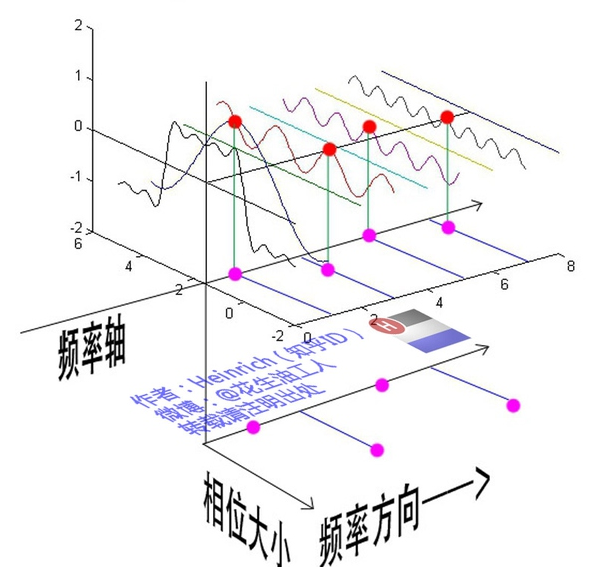F(u,v)=x=0M1y=0N1f(x,y)ej2π(ux/M+vy/N)

f(x,y)=1MNx=0M1y=0N1F(u,v)ej2π(ux/M+vy/N)

F(0,0)=x=0M1y=0N1f(x,y)=f¯(x,y)

import cv2
import numpy as np
import matplotlib.pyplot as plt

f = np.fft.fft2(img)
fshift = np.fft.fftshift(f)
#取绝对值：将复数变化成实数
#取对数的目的为了将数据变化到较小的范围（比如0-255）
s1 = np.log(np.abs(f))
s2 = np.log(np.abs(fshift))
plt.subplot(121),plt.imshow(s1,'gray'),plt.title('original')
plt.subplot(122),plt.imshow(s2,'gray'),plt.title('center')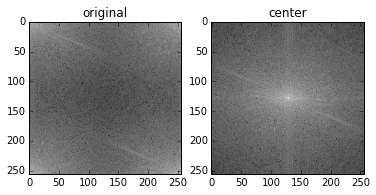import cv2
import numpy as np
import matplotlib.pyplot as plt

f = np.fft.fft2(img)
fshift = np.fft.fftshift(f)
#取绝对值：将复数变化成实数
#取对数的目的为了将数据变化到较小的范围（比如0-255）
ph_f = np.angle(f)
ph_fshift = np.angle(fshift)

plt.subplot(121),plt.imshow(ph_f,'gray'),plt.title('original')
plt.subplot(122),plt.imshow(ph_fshift,'gray'),plt.title('center')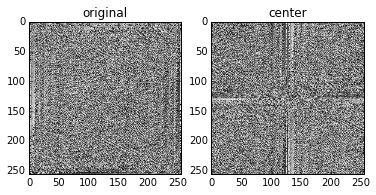Ok再来说说程序中为什么要有一个np.fft.fftshift(f)中心化操作，整个图像是在傅里叶变换的一个周期内完成的，将其看成横纵两个方向的一维傅里叶变换，在每个方向上都会有高频信号和低频信号，那么傅里叶变换将低频信号放在了边缘，高频信号放在了中间，然而一副图像，很明显的低频信号多而明显，所以将低频信号采用一种方法移到中间，在时域上就是对f乘以（-1）^(M+N)，换到频域里面就是位置的移到了。

import cv2
import numpy as np
import matplotlib.pyplot as plt

f = np.fft.fft2(img)
fshift = np.fft.fftshift(f)
#取绝对值：将复数变化成实数
#取对数的目的为了将数据变化到0-255
s1 = np.log(np.abs(fshift))
plt.subplot(131),plt.imshow(img,'gray'),plt.title('original')
plt.subplot(132),plt.imshow(s1,'gray'),plt.title('center')
# 逆变换
f1shift = np.fft.ifftshift(fshift)
img_back = np.fft.ifft2(f1shift)
#出来的是复数，无法显示
img_back = np.abs(img_back)
plt.subplot(133),plt.imshow(img_back,'gray'),plt.title('img back')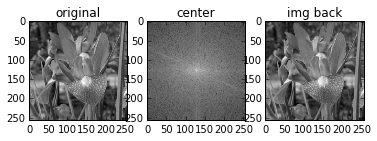import cv2
import numpy as np
import matplotlib.pyplot as plt

f = np.fft.fft2(img)
fshift = np.fft.fftshift(f)
#取绝对值：将复数变化成实数
#取对数的目的为了将数据变化到0-255
s1 = np.log(np.abs(fshift))
plt.subplot(221),plt.imshow(img,'gray'),plt.title('original')
plt.xticks([]),plt.yticks([])
#---------------------------------------------
# 逆变换--取绝对值就是振幅
f1shift = np.fft.ifftshift(np.abs(fshift))
img_back = np.fft.ifft2(f1shift)
#出来的是复数，无法显示
img_back = np.abs(img_back)
#调整大小范围便于显示
img_back = (img_back-np.amin(img_back))/(np.amax(img_back)-np.amin(img_back))
plt.subplot(222),plt.imshow(img_back,'gray'),plt.title('only Amplitude')
plt.xticks([]),plt.yticks([])
#---------------------------------------------
# 逆变换--取相位
f2shift = np.fft.ifftshift(np.angle(fshift))
img_back = np.fft.ifft2(f2shift)
#出来的是复数，无法显示
img_back = np.abs(img_back)
#调整大小范围便于显示
img_back = (img_back-np.amin(img_back))/(np.amax(img_back)-np.amin(img_back))
plt.subplot(223),plt.imshow(img_back,'gray'),plt.title('only phase')
plt.xticks([]),plt.yticks([])
#---------------------------------------------
# 逆变换--将两者合成看看
s1 = np.abs(fshift) #取振幅
s1_angle = np.angle(fshift) #取相位
s1_real = s1*np.cos(s1_angle) #取实部
s1_imag = s1*np.sin(s1_angle) #取虚部
s2 = np.zeros(img.shape,dtype=complex)
s2.real = np.array(s1_real) #重新赋值给s2
s2.imag = np.array(s1_imag)

f2shift = np.fft.ifftshift(s2) #对新的进行逆变换
img_back = np.fft.ifft2(f2shift)
#出来的是复数，无法显示
img_back = np.abs(img_back)
#调整大小范围便于显示
img_back = (img_back-np.amin(img_back))/(np.amax(img_back)-np.amin(img_back))
plt.subplot(224),plt.imshow(img_back,'gray'),plt.title('another way')
plt.xticks([]),plt.yticks([])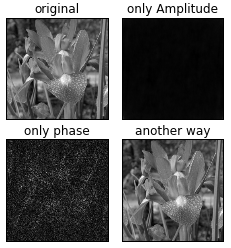import cv2
import numpy as np
import matplotlib.pyplot as plt

plt.subplot(221),plt.imshow(img_flower,'gray'),plt.title('origial1')
plt.xticks([]),plt.yticks([])
plt.subplot(222),plt.imshow(img_man,'gray'),plt.title('origial_2')
plt.xticks([]),plt.yticks([])
#--------------------------------
f1 = np.fft.fft2(img_flower)
f1shift = np.fft.fftshift(f1)
f1_A = np.abs(f1shift) #取振幅
f1_P = np.angle(f1shift) #取相位
#--------------------------------
f2 = np.fft.fft2(img_man)
f2shift = np.fft.fftshift(f2)
f2_A = np.abs(f2shift) #取振幅
f2_P = np.angle(f2shift) #取相位
#---图1的振幅--图2的相位--------------------
img_new1_f = np.zeros(img_flower.shape,dtype=complex)
img1_real = f1_A*np.cos(f2_P) #取实部
img1_imag = f1_A*np.sin(f2_P) #取虚部
img_new1_f.real = np.array(img1_real)
img_new1_f.imag = np.array(img1_imag)
f3shift = np.fft.ifftshift(img_new1_f) #对新的进行逆变换
img_new1 = np.fft.ifft2(f3shift)
#出来的是复数，无法显示
img_new1 = np.abs(img_new1)
#调整大小范围便于显示
img_new1 = (img_new1-np.amin(img_new1))/(np.amax(img_new1)-np.amin(img_new1))
plt.subplot(223),plt.imshow(img_new1,'gray'),plt.title('another way')
plt.xticks([]),plt.yticks([])
#---图2的振幅--图1的相位--------------------
img_new2_f = np.zeros(img_flower.shape,dtype=complex)
img2_real = f2_A*np.cos(f1_P) #取实部
img2_imag = f2_A*np.sin(f1_P) #取虚部
img_new2_f.real = np.array(img2_real)
img_new2_f.imag = np.array(img2_imag)
f4shift = np.fft.ifftshift(img_new2_f) #对新的进行逆变换
img_new2 = np.fft.ifft2(f4shift)
#出来的是复数，无法显示
img_new2 = np.abs(img_new2)
#调整大小范围便于显示
img_new2 = (img_new2-np.amin(img_new2))/(np.amax(img_new2)-np.amin(img_new2))
plt.subplot(224),plt.imshow(img_new2,'gray'),plt.title('another way')
plt.xticks([]),plt.yticks([])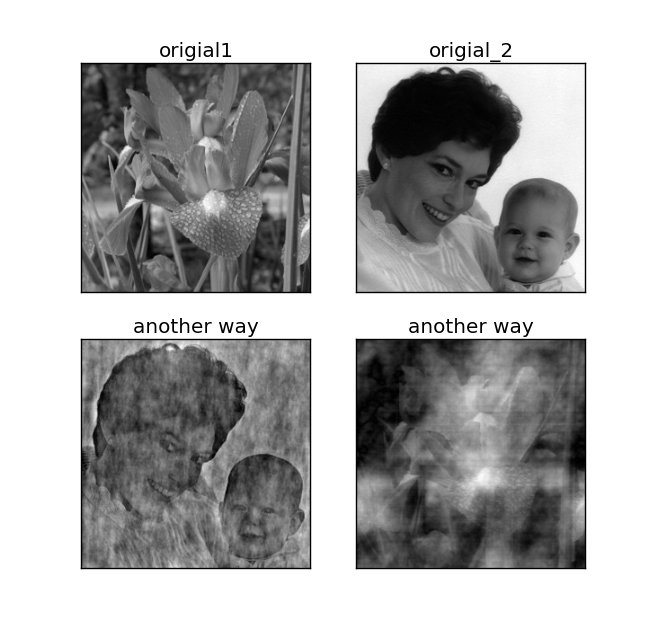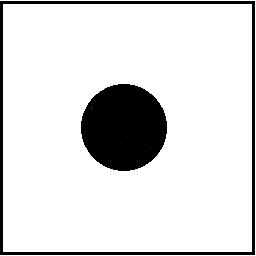import cv2
import numpy as np
import matplotlib.pyplot as plt

plt.subplot(121),plt.imshow(img_man,'gray'),plt.title('origial')
plt.xticks([]),plt.yticks([])
#--------------------------------
rows,cols = img_man.shape
#--------------------------------
f1 = np.fft.fft2(img_man)
f1shift = np.fft.fftshift(f1)
f2shift = np.fft.ifftshift(f1shift) #对新的进行逆变换
img_new = np.fft.ifft2(f2shift)
#出来的是复数，无法显示
img_new = np.abs(img_new)
#调整大小范围便于显示
img_new = (img_new-np.amin(img_new))/(np.amax(img_new)-np.amin(img_new))
plt.subplot(122),plt.imshow(img_new,'gray'),plt.title('Highpass')
plt.xticks([]),plt.yticks([])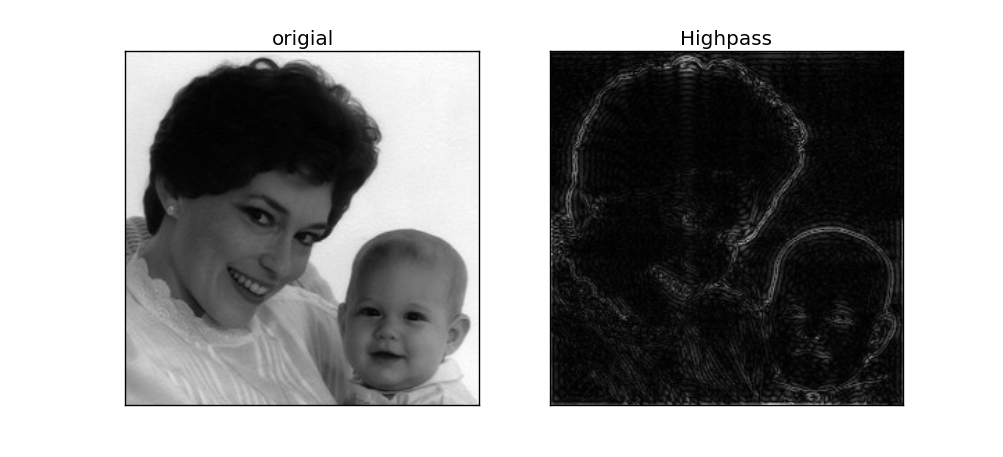M=010141010

import numpy as np
import matplotlib.pyplot as plt

laplacian = np.array([[0,1,0],[1,-4,1],[0,1,0]])
f = np.fft.fft2(laplacian)
f1shift = np.fft.fftshift(f)
img = np.log(np.abs(f1shift))
plt.imshow(img,'gray')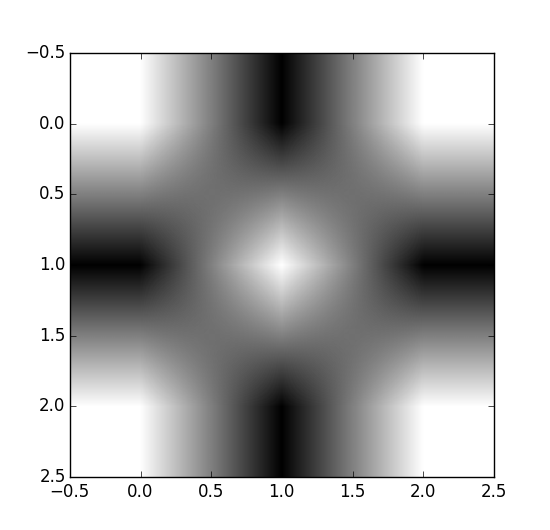import cv2
import numpy as np
import matplotlib.pyplot as plt

plt.subplot(121),plt.imshow(img_man,'gray'),plt.title('origial')
plt.xticks([]),plt.yticks([])
#--------------------------------
rows,cols = img_man.shape
#--------------------------------
f1 = np.fft.fft2(img_man)
f1shift = np.fft.fftshift(f1)
f2shift = np.fft.ifftshift(f1shift) #对新的进行逆变换
img_new = np.fft.ifft2(f2shift)
#出来的是复数，无法显示
img_new = np.abs(img_new)
#调整大小范围便于显示
img_new = (img_new-np.amin(img_new))/(np.amax(img_new)-np.amin(img_new))
plt.subplot(122),plt.imshow(img_new,'gray'),plt.title('Highpass')
plt.xticks([]),plt.yticks([])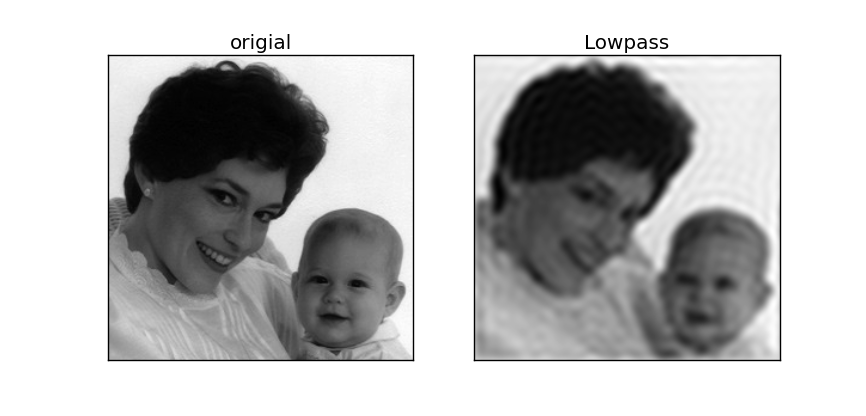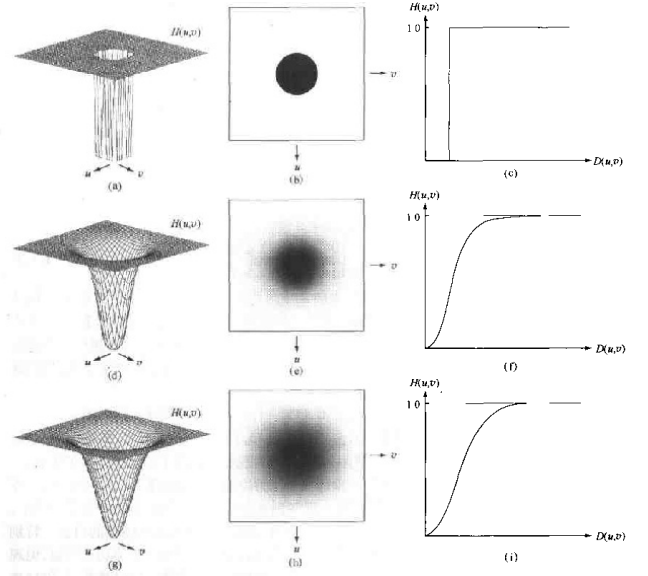import cv2
import numpy as np
import matplotlib.pyplot as plt

plt.subplot(121),plt.imshow(img_man,'gray'),plt.title('origial')
plt.xticks([]),plt.yticks([])
#--------------------------------
rows,cols = img_man.shape
#--------------------------------
f1 = np.fft.fft2(img_man)
f1shift = np.fft.fftshift(f1)
f2shift = np.fft.ifftshift(f1shift) #对新的进行逆变换
img_new = np.fft.ifft2(f2shift)
#出来的是复数，无法显示
img_new = np.abs(img_new)
#调整大小范围便于显示
img_new = (img_new-np.amin(img_new))/(np.amax(img_new)-np.amin(img_new))
plt.subplot(122),plt.imshow(img_new,'gray')
plt.xticks([]),plt.yticks([])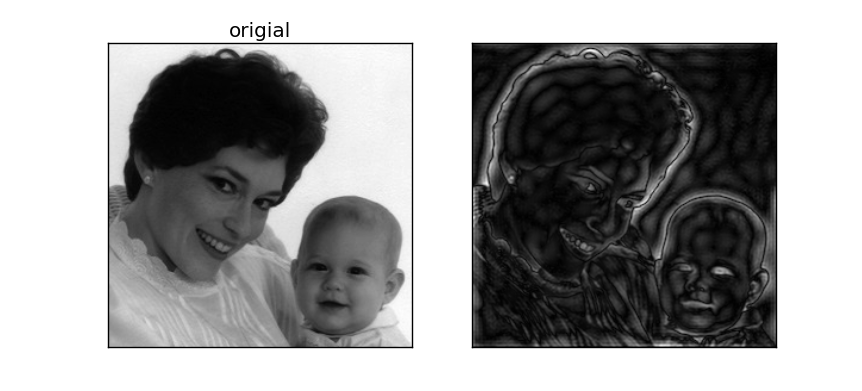05-292777
06-15171
12-25183
02-075181
08-16261
01-271082
02-074430
06-256万+
07-111101